Related Articles

# Clockwise/Spiral Rule in C/C++ with Examples

• Difficulty Level : Medium
• Last Updated : 22 Sep, 2021

The Spiral/Clockwise Method is a magic tool for C/C++ programmers to define the meaning of syntax declaration in the head within seconds. This method was created by David Anderson and here is a short brief about how to apply this method.

While coding, when someone comes across a new unwanted syntax declaration:

`string(const** f(int, void (*const p)(int)))(char[]);`

The question here arises is what is the meaning of the function name “f”? There is an infamous way that will help one to deduce the meaning of this function “f” in seconds.

Approach:

Step 1: Consider this Clockwise-spiral.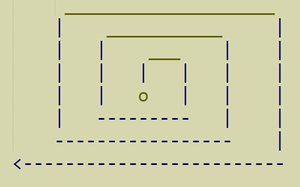Step 2: Replace O with a.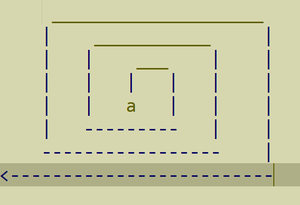Step3: Now, along the clockwise path, replace “|” with some words and try to get the meaning of syntax, and replace “|” with “(“. The round brackets explain its function and say “‘a’ is a function”.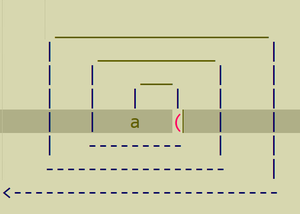Step 4: Consider seeing what is inside of the round brackets/function, so the open brackets (remember like from precedence of operators, brackets are always opened first) and go from left to right until the end of round brackets  “)” are encountered. It can be said that “‘a’ is a function with the integer ‘x’ as an argument”.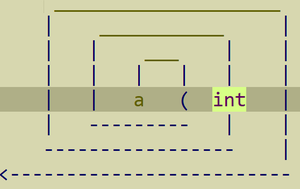Step 5: Now come back to the first round bracket and continue the spiral path from “(“. Replace “|” with “*”. This means a return value and pointer. Here “‘a’ is a function with the integer ‘x’ as an argument which returns a pointer to “.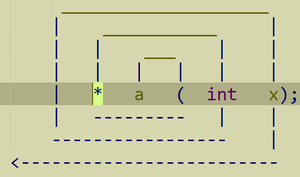Step 6: Continue the spiral clockwise. One will encounter “int” which was already dealt with, so continue the spiral path.Step 7: Replace “|” with “int”. It can be said that “‘a’ is a function with the integer ‘x’ as an argument which returns a pointer to an integer”.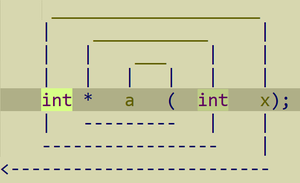Step 8: Again, continue this path and one will get “;” which means this is the end of the statement, and see that’s how the meaning of syntax can be defined easily.Finally, it can be said that “‘a’ is a function with an integer ‘x’ as an argument which returns a pointer to the integer”.

Answer: f is a function with arguments as int and p a function with the argument as int, returning a constant pointer to nothing (void) returning a pointer to a pointer to a constant function with the character array as an argument returning a string.

Important Points:

1. Syntax Explanations:
• A[ ]: array A is of undefined size and A[ 5 ] means the size of array is 5.
• (type 1,type 2): function passing type1 and type2.
• *: pointer to.
3. Keep this reading until all modifiers in the variable declaration are overwritten.
4. Always complete any content in parentheses first.
5. Keep doing this in a spiral/clockwise direction until all tokens have been covered.

Example:
Consider an example by visualizing a spiral in the brain:

`(char const *p) ( int , const (int  *)*);`

Remember the thumb rule. Always start with the name of a variable. Starting from a variable p clockwise spiral.

Step 1: ‘p’ is the variable.

`p                                              `

Step 2: ‘p’ is a variable that is a pointer to.

`p -> *                                     `

Step 3: ‘p’ is a variable that is a pointer to a function.

`p -> *  -> (                             `

Step 4: p’ is a variable that is a pointer to a function with int as an argument.

`p -> *  -> (  -> int               `

Step 5: For the next step, there is no variable name, so give one. Let it be ‘a’. So the expression can be written as:

`(char const *p) ( int , const (int  *)* a );`

Step 6: Here ‘a’ is a variable.

`a     `

Step 7: Closed round bracket doesn’t tell anything, so continue.

`a-> )                          `

Step 8: In the next step, a is a variable pointer to.

`a -> ) -> *      `

Step 9: Ignore the semicolon because the syntax definition is not complete, so continue.

`a -> ) -> *  -> ;  `

Step 10: The closed round bracket doesn’t tell anything, so continue.

`a -> ) -> *  -> ; -> )`

Step 11: Now ‘a’ is a variable pointer to pointer.

`a -> ) -> *  -> ; -> ) -> *                                                                      `

Step 12: ‘a’ is a variable pointer to pointer int.

`a -> ) -> *  -> ; -> ) -> * -> int                     `

Step 13: ‘a’ is a variable pointer to pointer int constant.

`a -> ) -> *  -> ; -> ) -> * -> int -> const     `

Let’s get back to where defining ‘p’ was left.

Step 14: ‘p’ is a variable that is a pointer to a function with int as an argument and a variable pointer to the pointer int constant which returns a constant.

`p -> *  -> (  -> int , const... -> const`

Note:
The reused terms already used for defining syntax are being ignored here.

Step 15: p is a variable that is a pointer to a function with int as argument and a variable pointer to pointer int constant which returns a constant character.

`p -> *  -> (  -> int , const... -> const->char`

This finishes the complete meaning of the syntax.

Note:
There is an exception to this rule when syntax has arrays (especially multidimensional arrays), so there is an update to this rule.

• When an array is encountered, move to the most-right closing square bracket and treat the array as one ‘sweep’ “
• Example:
• int *a[]: ‘a’ is a multidimensional array of size 10 and an undefined size of pointers to int.
Want to learn from the best curated videos and practice problems, check out the C++ Foundation Course for Basic to Advanced C++ and C++ STL Course for foundation plus STL.  To complete your preparation from learning a language to DS Algo and many more,  please refer Complete Interview Preparation Course.

My Personal Notes arrow_drop_up